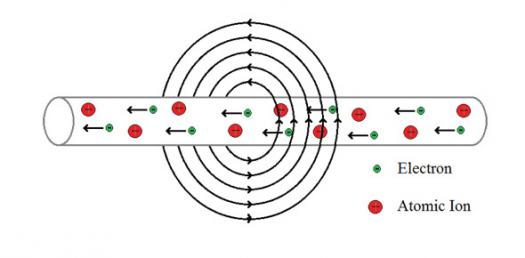# Electromagnetism

10 kysymyst� | Total Attempts: 1084Settings.

Related Topics
• 1.
What is an Alternating Current?
• A.

An electric Current that changes its direction.

• B.

An Electric Charge that doesn't change its path.

• C.

An electromagnetic wave.

• D.

None of the above

• 2.
What is a Domain composed of?
• A.

Atoms

• B.

Energy

• C.

Electricity

• D.

None of the above

• 3.
An electric motor converts  electrical energy to __________ energy
• A.

Potential

• B.

Kinetic

• C.

Both a and b

• D.

None of the above

• 4.
What is the power level of an Electromagnet?
• A.

Strong

• B.

Weak

• C.

Medium

• D.

Nuclear

• 5.
What is Electromagnetic Induction?
• A.

The production of a current in a circuit by a change in strength.

• B.

Region where a force can be detected

• C.

Both a and b

• D.

None of the above

• 6.
What does a Galvanometer measure?
• A.

The amount of current in a circuit

• B.

The amount of votage in a circuit

• C.

The amount of electrical power in a region

• D.

None of the above

• 7.
What does a generator switch?
• A.

Energy types

• B.

Direction

• C.

Both a and b

• D.

None of the above

• 8.
What is a magnetic field?
• A.

An area where a magnetic force can be detected

• B.

Where you play magnetic ball

• C.

Both a and b

• D.

None of the above

• 9.
What is a solenoid?
• A.

A coil of insulated wire

• B.

An asteroid in outer space

• C.

Both a and b

• D.

None of the above

• 10.
What does a transformer change?
• A.

Voltage

• B.

Mass

• C.

Weight

• D.

None of the above